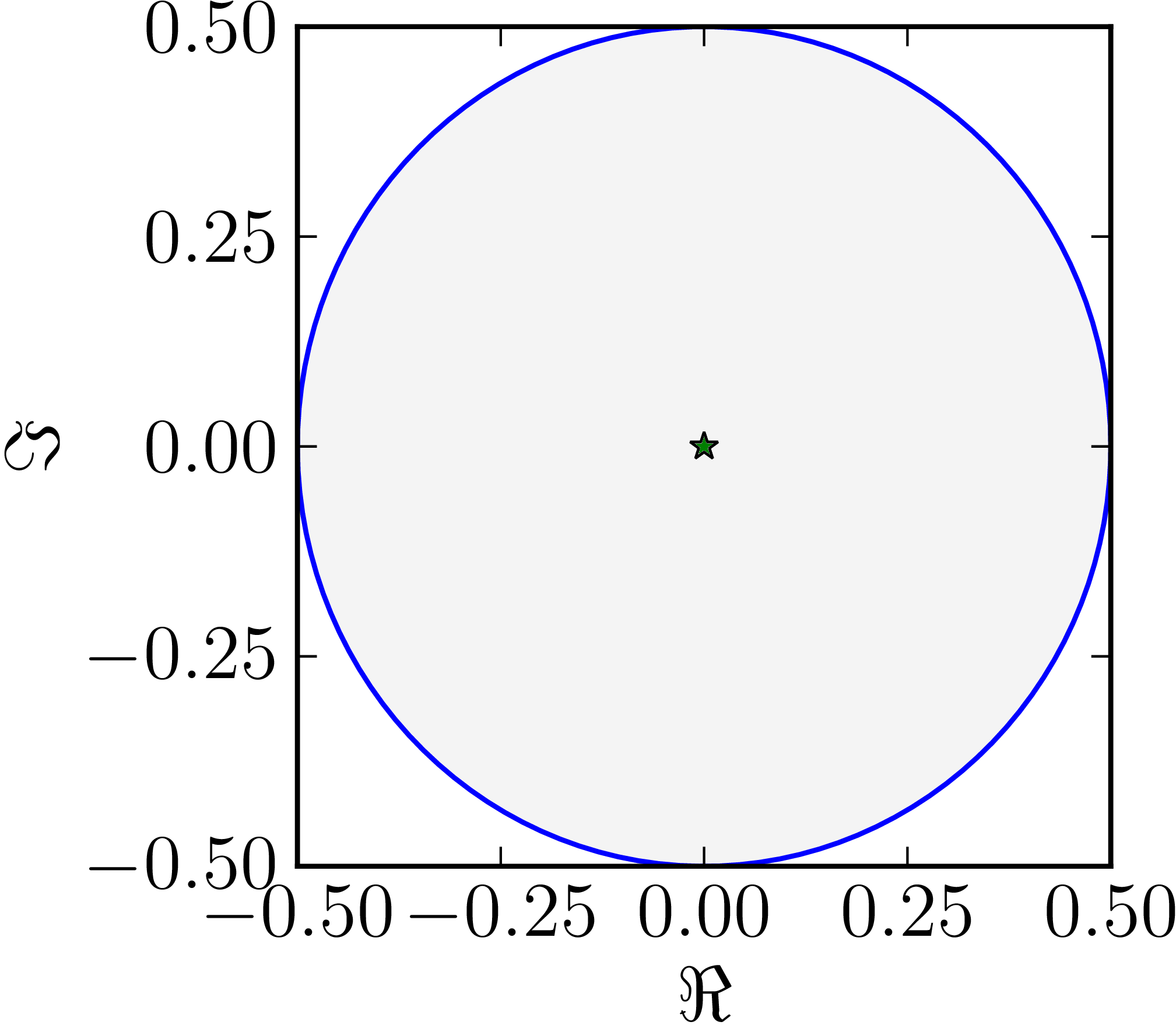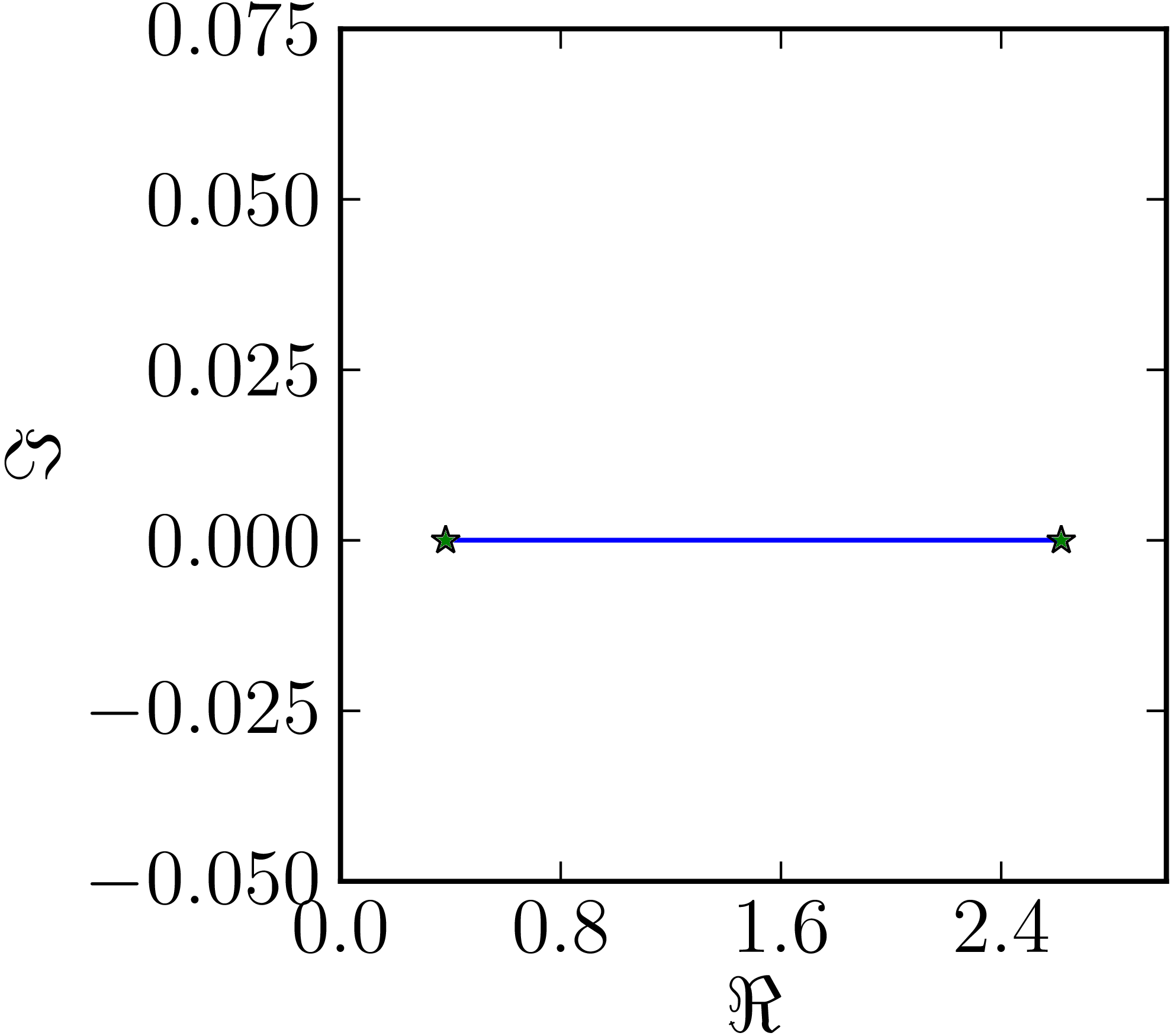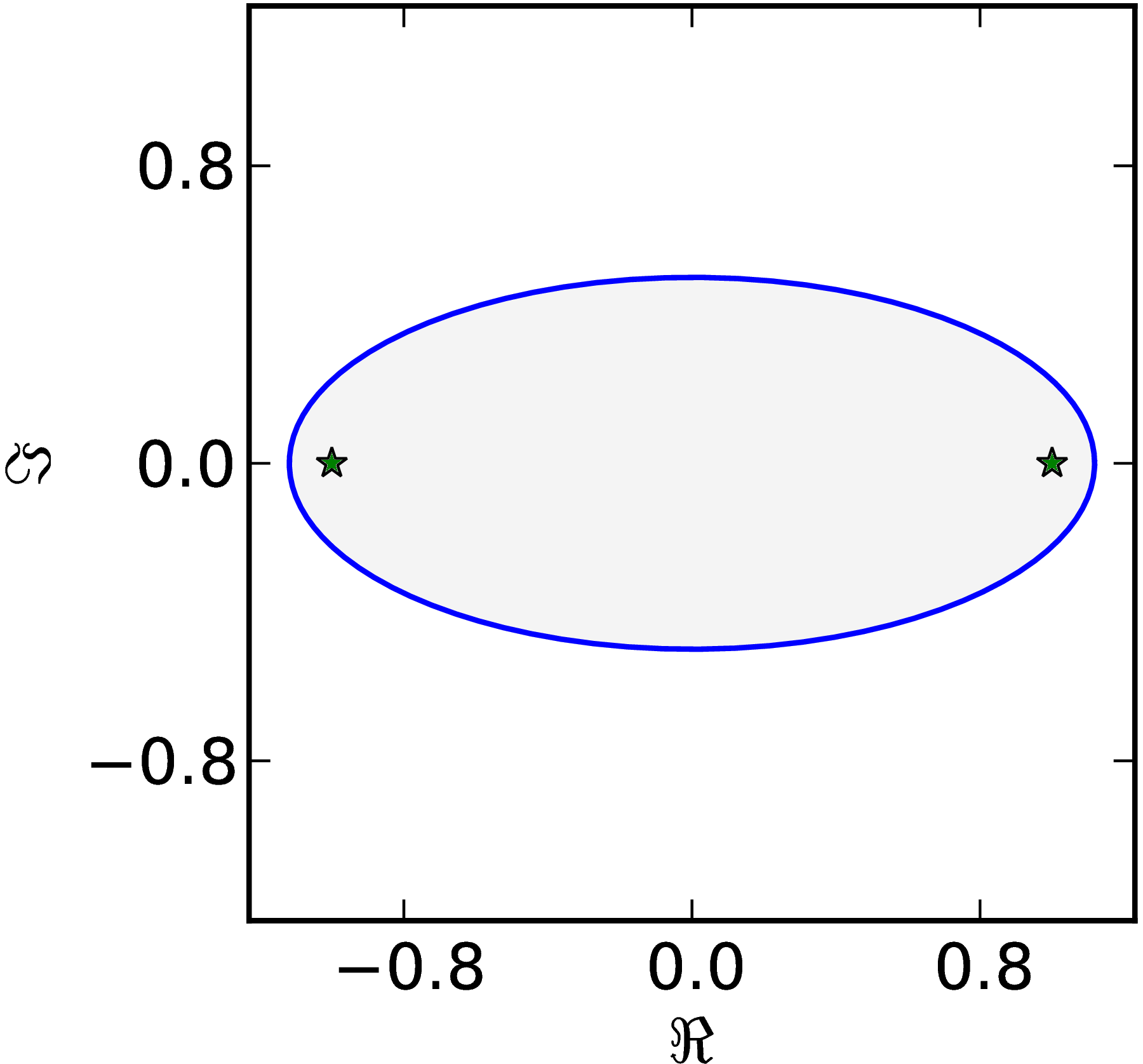### Example 1

• For a Jordan matrix $J=\begin{pmatrix}0&1\\0&0\end{pmatrix}$ the numerical range forms a circular disk of radius one half, $W(J) = D(0,)$.
Example of a circular numerical range.### Example 2

• Normal matrix of order $d=2$: the disk reduces to an interval $[\lambda_1, \lambda_2]$ in the complex plane. The matrix in the example is $M=\begin{pmatrix}1&1\ii\\-1\ii&2\end{pmatrix}$
Numerical range of a normal matrix.### Example 3

• Generic matrix of order $d=2$ the numerical range $W(M)$ is an ellipse. The foci of the ellipse are located in the eigenvalues. The matrix in the example is $M=\begin{pmatrix}1&1\\0&-1\end{pmatrix}$.
Numerical range of a non-normal matrix.